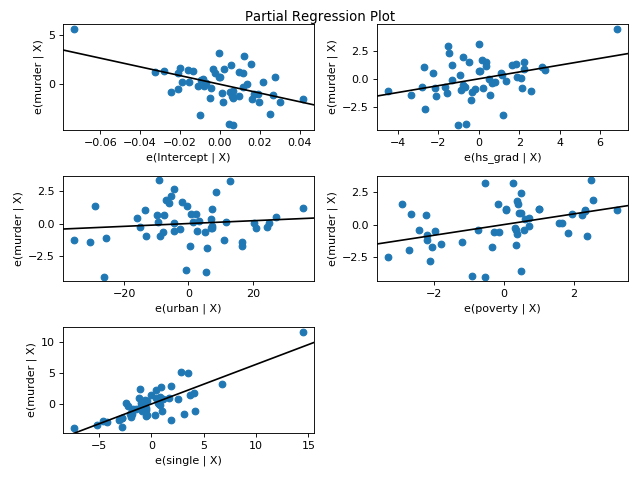# statsmodels.graphics.regressionplots.plot_partregress_grid¶

statsmodels.graphics.regressionplots.plot_partregress_grid(results, exog_idx=None, grid=None, fig=None)[source]

Plot partial regression for a set of regressors.

Parameters
resultsresults instance

A regression model results instance

exog_idxNone, list of ints, list of strings

(column) indices of the exog used in the plot, default is all.

gridNone or tuple of int (nrows, ncols)

If grid is given, then it is used for the arrangement of the subplots. If grid is None, then ncol is one, if there are only 2 subplots, and the number of columns is two otherwise.

figMatplotlib figure instance, optional

If given, this figure is simply returned. Otherwise a new figure is created.

Returns
figMatplotlib figure instance

If fig is None, the created figure. Otherwise fig itself.

plot_partregress

Plot partial regression for a single regressor.

plot_ccpr

Plot CCPR against one regressor

Notes

A subplot is created for each explanatory variable given by exog_idx. The partial regression plot shows the relationship between the response and the given explanatory variable after removing the effect of all other explanatory variables in exog.

References

Examples

Using the state crime dataset seperately plot the effect of the each variable on the on the outcome, murder rate while accounting for the effect of all other variables in the model visualized with a grid of partial regression plots.

>>> from statsmodels.graphics.regressionplots import plot_partregress_grid
>>> import statsmodels.api as sm
>>> import matplotlib.pyplot as plt
>>> import statsmodels.formula.api as smf

>>> fig = plt.figure(figsize=(8, 6))